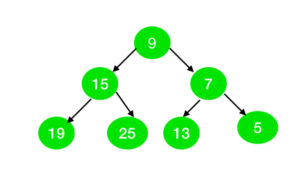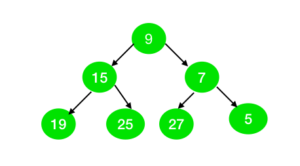# Check if all nodes of the Binary Tree can be represented as sum of two primes

Given a binary tree of N nodes with odd value. The task is to check whether all the nodes of the tree can be represented as the sum of the two prime numbers or not.

Examples:

Input:Output: Yes
Explanation:
All the nodes in the tree can be represented as the sum of two prime numbers as:
9 = 2 + 7
15 = 2 +13
7 = 2 + 5
19 = 2 + 17
25 = 2 + 23
13 = 11 + 2
5 = 2 + 3

Input:Output: No
Explanation:
The node with value 27 cannot be represented as the sum of two prime numbers.

Approach:

1. The idea is to use Goldbach’s Weak Conjecture which states that every odd number greater than 5 can be expressed as the sum of three primes.
2. To represent odd number(say N) as a sum of two prime numbers, fix one prime number as 2 and if (N – 2) is also prime, then N can be represented as a sum of two prime numbers.
3. Check the above condition for all the nodes in a tree. If any node doesn’t follow the above condition then print “No” else print “Yes”.

 `// C++ program for the above approach ` `#include ` `using` `namespace` `std; ` ` `  `// Function to create array to mark ` `// whether element are prime or not ` `void` `spf_array(``int` `arr[], ``int` `N) ` `{ ` `    ``int` `i = 0; ` ` `  `    ``// Initially we set same value in ` `    ``// array as a index of array. ` `    ``for` `(i = 1; i <= N; i++) { ` `        ``arr[i] = i; ` `    ``} ` ` `  `    ``// Mark all even elements as 2 ` `    ``for` `(i = 2; i <= N; i = i + 2) { ` `        ``arr[i] = 2; ` `    ``} ` ` `  `    ``// Mark all the multiple of prime ` `    ``// numbers as a non-prime ` `    ``for` `(i = 3; i * i <= N; i++) { ` `        ``if` `(arr[i] == i) { ` ` `  `            ``int` `j = 0; ` ` `  `            ``for` `(j = i * i; j <= N; ` `                 ``j = j + i) { ` ` `  `                ``if` `(arr[j] == j) { ` `                    ``arr[j] = i; ` `                ``} ` `            ``} ` `        ``} ` `    ``} ` `} ` ` `  `// Tree Node ` `struct` `node { ` `    ``int` `val; ` `    ``node* left; ` `    ``node* right; ` `}; ` ` `  `// Funtion to create node of tree ` `node* newnode(``int` `i) ` `{ ` `    ``node* temp = NULL; ` `    ``temp = ``new` `node(); ` `    ``temp->val = i; ` `    ``temp->left = NULL; ` `    ``temp->right = NULL; ` `    ``return` `temp; ` `} ` ` `  `// Function to check whether the ` `// tree is prime or not ` `int` `prime_tree(node* root, ``int` `arr[]) ` `{ ` `    ``int` `a = -1; ` `    ``if` `(root != NULL) { ` ` `  `        ``// If element is not the sum of ` `        ``// two prime then return 0 ` `        ``if` `(root->val <= 3 ` `            ``|| arr[root->val - 2] ` `                   ``!= root->val - 2) { ` ` `  `            ``return` `0; ` `        ``} ` `    ``} ` ` `  `    ``if` `(root->left != NULL) { ` `        ``a = prime_tree(root->left, arr); ` ` `  `        ``// If a is 0 then we don't need ` `        ``// to check further ` `        ``if` `(a == 0) { ` `            ``return` `0; ` `        ``} ` `    ``} ` ` `  `    ``if` `(root->right != NULL) { ` ` `  `        ``a = prime_tree(root->right, arr); ` ` `  `        ``// If a is 0 then we don't need ` `        ``// to check further ` `        ``if` `(a == 0) { ` `            ``return` `0; ` `        ``} ` `    ``} ` ` `  `    ``return` `1; ` `} ` ` `  `// Driver Code ` `int` `main() ` `{ ` ` `  `    ``// GIven Tree ` `    ``node* root = newnode(9); ` `    ``root->right = newnode(7); ` `    ``root->right->right = newnode(5); ` `    ``root->right->left = newnode(13); ` `    ``root->left = newnode(15); ` `    ``root->left->left = newnode(19); ` `    ``root->left->right = newnode(25); ` ` `  `    ``// Number of nodes in the tree ` `    ``int` `n = 50; ` ` `  `    ``// Declare spf[] to store ` `    ``// prime numbers ` `    ``int` `brr[n + 1]; ` `    ``int` `i = 0; ` ` `  `    ``// Find prime numbers in spf[] ` `    ``spf_array(brr, n + 1); ` ` `  `    ``// Function Call ` `    ``if` `(prime_tree(root, brr)) { ` `        ``cout << ``"Yes"` `<< endl; ` `    ``} ` `    ``else` `{ ` `        ``cout << ``"No"` `<< endl; ` `    ``} ` `} `

Output:

```Yes
```

Time complexity : O (N * log(log N))
Auxiliary Space : O (N)

Don’t stop now and take your learning to the next level. Learn all the important concepts of Data Structures and Algorithms with the help of the most trusted course: DSA Self Paced. Become industry ready at a student-friendly price.

My Personal Notes arrow_drop_upCheck out this Author's contributed articles.

If you like GeeksforGeeks and would like to contribute, you can also write an article using contribute.geeksforgeeks.org or mail your article to contribute@geeksforgeeks.org. See your article appearing on the GeeksforGeeks main page and help other Geeks.

Please Improve this article if you find anything incorrect by clicking on the "Improve Article" button below.